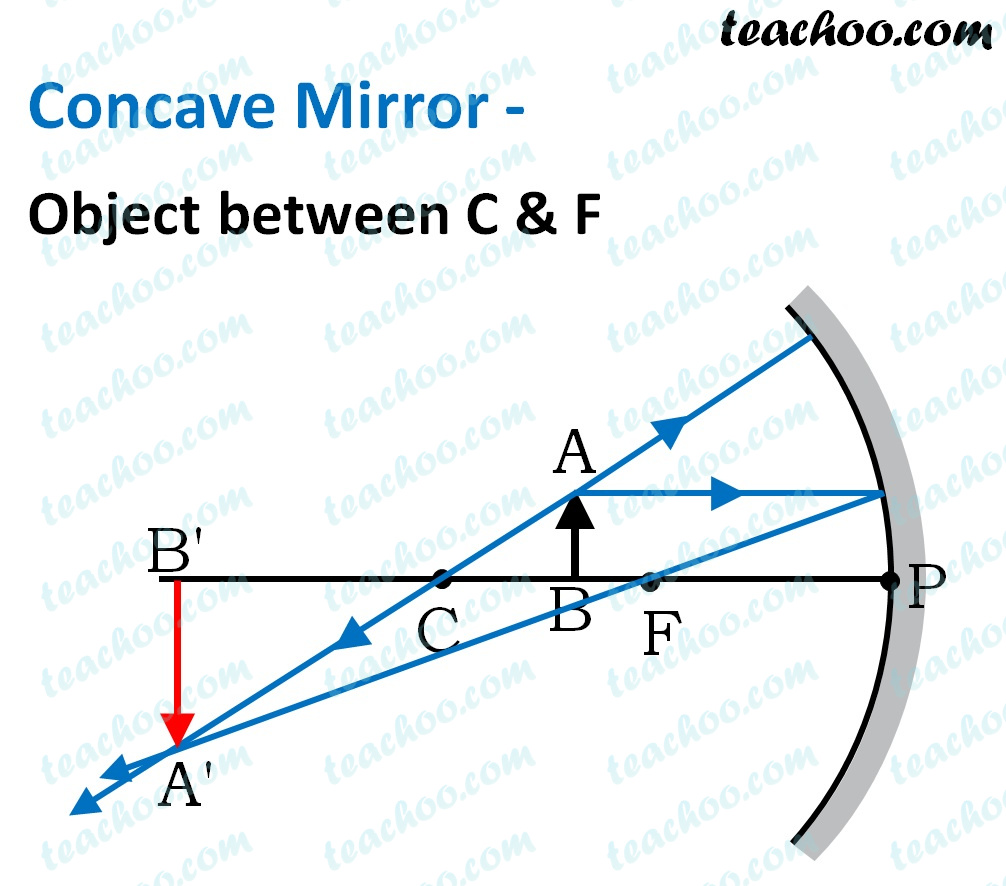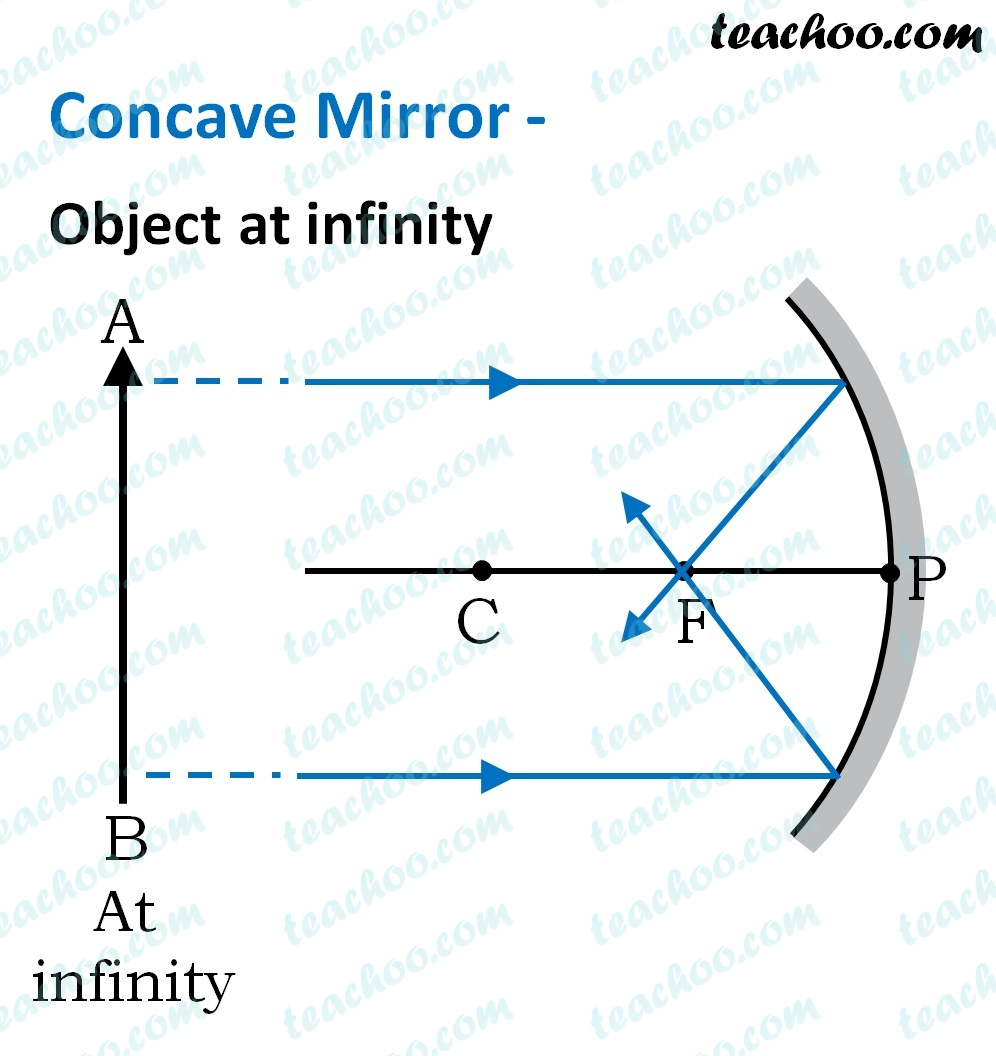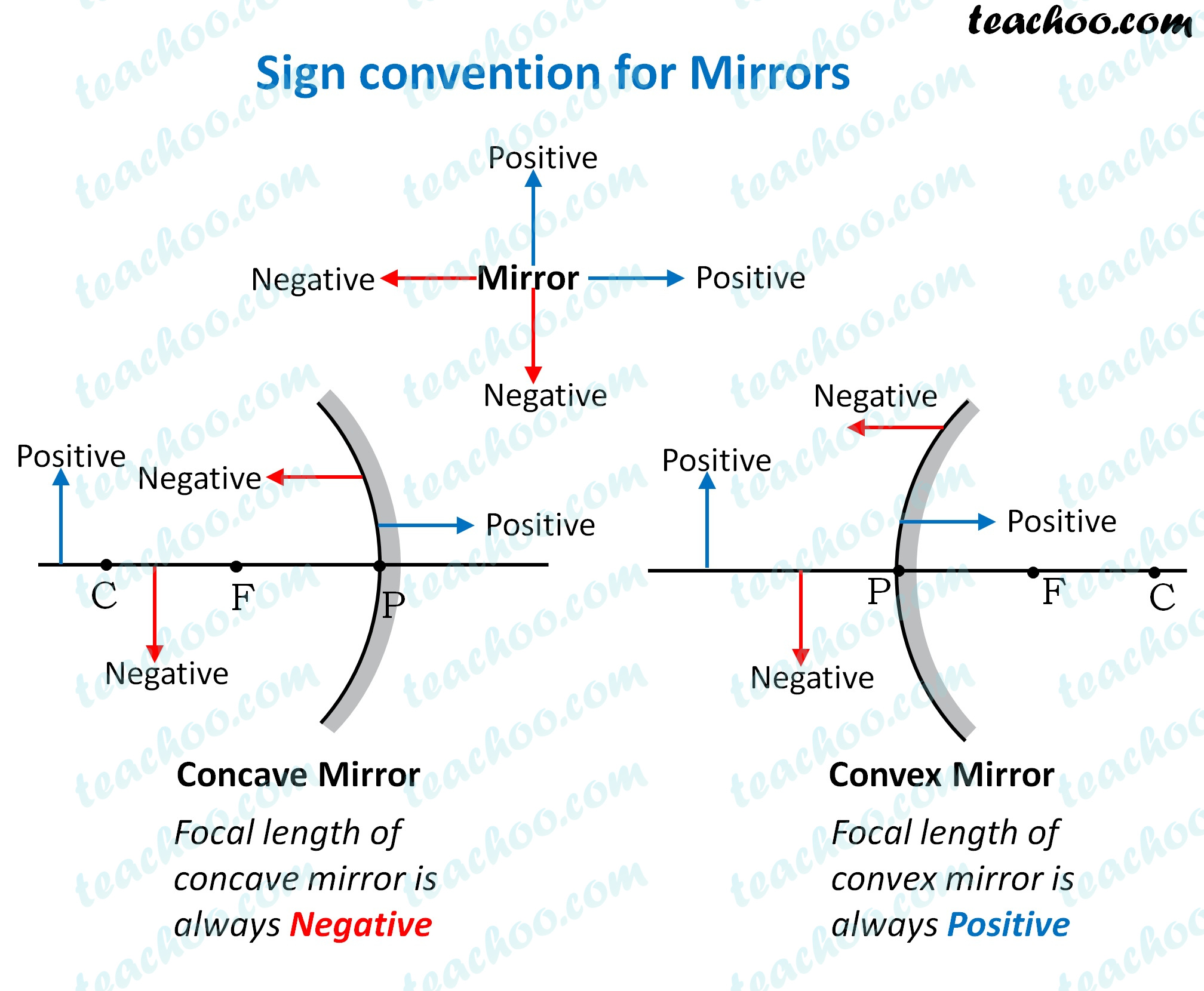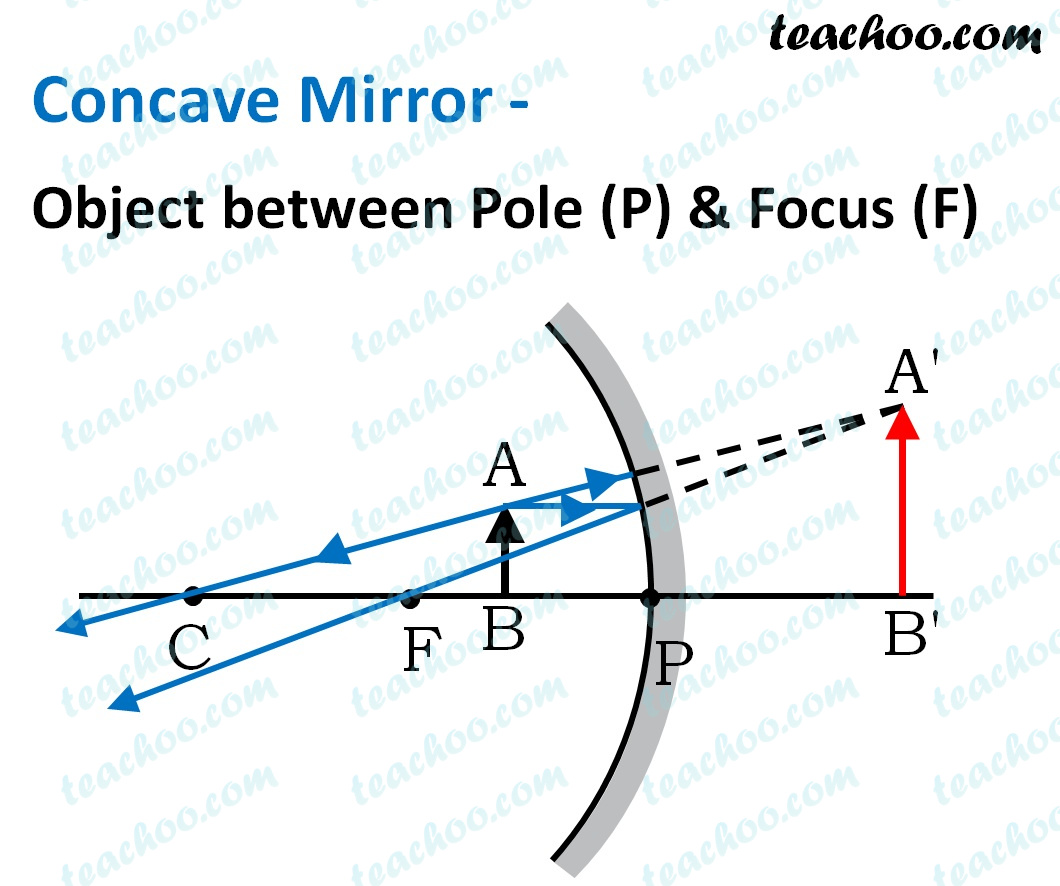Case Based Questions (MCQ)

Class 10
Chapter 10 Class 10 - Light - Reflection and Refraction (Term 1)

## A student wants to project the image of a candle  flame on the walls of the school laboratory by  using a mirror.

### (iv) Convex mirror, it forms real image

Ans.

Since the student wants image on a wall , it has to be a real image

Now, let’s check both mirrors

• Convex mirrors always produce virtual images . It cannot be used to produce images on the screen.
• Concave mirrors produce real images on the screen in all cases except when the object is between pole and focus , therefore students should use a concave mirror because it forms real images.

So, the correct answer is (iii) Concave mirror, it forms a real image.

### (iv) At infinity

Ans.

In a concave mirror

• We get a magnified and real image when object is placed between F and CSo, the correct answer is (ii) Between F and C

### (iv) At F

Ans.

For a concave mirror

• When rays are coming from infinity , a concave mirror will produce a diminished image on the screen.And for other cases - please check Concave mirror ray diagram

So, the correct answer is (i) at infinity

## (iv) None of the above

Ans.

According to the Cartesian sign convention for spherical mirrors, images formed on the left side of the mirror are signed as negative .So, the correct answer is (ii) negative

## (iv) inverted and enlarged

Ans.
For a concave mirror

• Virtual image is formed when an object is placed between pole and focus .
• And, Image formed is enlarged .So, the correct answer is (i) erect and enlarged

983 students joined Teachoo Black. What are you waiting for?